Next: 15. The Iota Constructor Up: 2. User's Guide Previous: 13. Simplification

Subsections

# 14. Macetes

In addition to its simplifier, IMPS also provides a separate mechanism for manipulating formal expressions by applying theorems. Unlike simplification, it is under the control of the user. The basic idea is that some expression manipulation procedures--for instance, conditional rewrite rules--are in fact direct applications of theorems. Other expression manipulation procedures can be formed from existing ones using a simple combination language. These expression manipulation procedures are called macetes.14.1 Formally, a macete is a function M which takes as arguments a context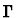and an expression e (called the source expression) and returns another expression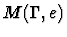(called the replacement expression). In general, the replacement expression does not have to be equal to the source expression.

Macetes serve two somewhat different purposes:

• They are a straightforward way to apply a theorem or a collection of theorems to a sequent in a deduction graph.
• They are also a mechanism for computing with theorems.

# 14.1 Classification

A macete can be atomic or compound. Compound macetes are obtained by applying a macete constructor to a list of one or more macetes.

Atomic macetes are classified as:

• Schematic macetes. A schematic macete is one in which the replacement expression is obtained from the source expression by matching and substitution. Schematic macetes can be thought of as conditional rewrite rules.
• Procedural macetes. These are macetes which compute the replacement expression by a more complicated procedure. There are only a few such procedures:
• simplify
• beta-reduce
• beta-reduce-insistently
Having these procedures as macetes allows you to build more powerful and useful compound macetes.

Macetes can be also classified according to the relation between the source and replacement expressions.

• Bidirectional. Whatever the context and source expression, the replacement expression is quasi-equal to the source expression, that is, given any legitimate model in which the context assumptions are true, either both source expression and replacement expression have no value or their value is identical.
• Backward-directional. Whatever the context and source expression, the replacement expression is quasi-equal to or implies the source expression. Notice that implication only makes sense for formulas of sort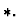• Nondirectional. There are contexts and source expressions in which the target expression is neither quasi-equal to nor implies the source expression.

# 14.2 Atomic Macetes

## 14.2.1 Schematic Macetes

A schematic macete can be associated to any formula in two ways depending on the kind of matching procedure used. IMPS has two general forms of matching called expression matching and translation matching.

Let us consider first the case of expression matching. To any IMPS formula we can associate:

• A source pattern. This is an expression s.
• A replacement pattern. This is an expression r.
• A list of condition patterns. This is a list of expressions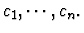• A direction specifier: this specifier can be either bidirectional or backward-directional.
Suppose the formula is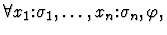where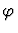itself is not a universal formula. The patterns are extracted fromdepending on the form ofas described by the following table:

 Form Source Replacement Conditions Spec.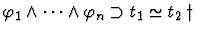t1 t2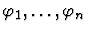Bid.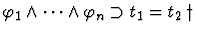t1 t2Bid.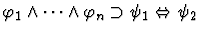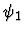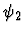Bid.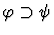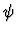None Back.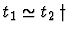t1 t2 None Bid.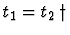t1 t2 None Bid.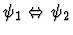None Bid.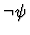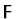None Bid.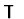None Bid.

Notes

• If the replacement patternaccording to the above table is of sort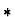and contains free variables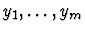not occurring freely in the source pattern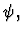then consider instead the replacement pattern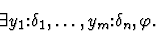• Nested implications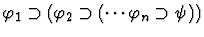are treated as single implications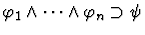when applying the above table.
• In those cases marked with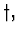if t2 contains free variables which do not occur freely in t1, then consider instead the source pattern t1 = t2 or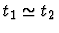and replacement pattern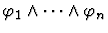or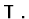We now describe how a schematic macete M is obtained from the source, replacement, condition patterns, and direction specifier of a formula. Given a contextand an expression t, the result of applying M toand t is determined as follows: Let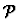be the set of paths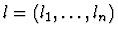to subexpressions elof t which satisfy all of the following conditions:

• If the directional specifier is backward-directional, the parity of lmust be +1; otherwise, no condition is imposed on the path.
• The source pattern s matches el. Thus there is a substitution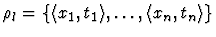such that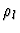applied to s gives el.
• The validity of all the convergence requirements of the substitutionand all the formulas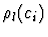in the contextlocalized at the path l are called the minor premises generated by the macete application. These formulas may or may not generate applicability conditions:
• If the macete is being applied with the accumulation of minor premises, then these formulas are posted as new sequents and must be proven separately. No new applicability conditions are generated in this case.
• Otherwise, the minor premises must be recognized as valid by the simplifier.
• No smaller path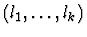satisfies the preceding conditions.
The replacement formula is obtained by replacing each subexpression el by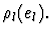Macetes obtained in this way are called elementary macetes.

Whenever you enter a theorem into a theory, the associated schematic macete is automatically installed, so that you can use it in deductions by hitting the key m.

The case of translation matching is similar, but instead of considering only convergence requirements, we need to insure that the translation component of the translation match is a theory interpretation. Macetes obtained in this way are called transportable macetes. The use of a theorem as a transportable macete must be entered into its usage list when the theorem is entered.

## 14.2.2 Nondirectional Macetes

Frequently it is useful to construct a schematic macete from an arbitrary formula by using a matching and substitution procedure which does not obey the variable capturing protections and other restrictions of normal matching. We refer to this as nullary matching and substitution. For instance, to apply a theorem which involves quantification over functions, one often has to build a lambda-abstraction of a sub-expression. We shall see later on how this can be done.

# 14.3 Compound Macetes

Compound macetes are built from atomic macetes and other compound macetes using macete constructors. The constructors are:

• Series: This constructor takes a list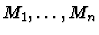of macetes as arguments and yields a macete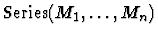which applies every macete in the list once, from left to right. Thus given a contextand an expression e,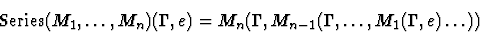• Sequential: This constructor takes any number of macetes as arguments. It works like the series constructor, except that when a macete fails to change an expression, subsequent macetes on the list are not applied.
• Parallel: This constructor takes a listof macetes as arguments and yields a macete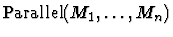which applies macetes in the list from left to right until a macete changes the expression. Thus given a contextand an expression e,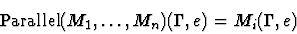provided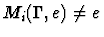and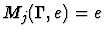for all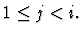• Repeat: Takes any number of macetes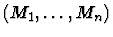as arguments and yields a macete in the following way: The series composition M ofis applied repeatedly to the expression e until no more change occurs. It may possibly compute forever. Thus the macete terminates if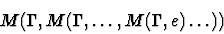stabilizes to some expression t. This expression is the value of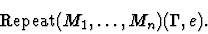• Sound: This constructor takes a list M1,M2,M3 of three macetes and yields a macete characterized as follows: Given a contextand an expression e,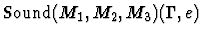is
•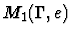if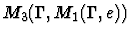is alpha-equivalent to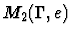• e otherwise.
• Without-minor-premises: This constructor takes a single argument macete M. It returns a new macete which applies M without minor premises. That is, macete substitution is only done when the macete requirements are satisfied.

Now whether the resulting macete is bidirectional or backward-directional, can be determined by considering the bidirectionality or backward-directionality each of the components.

• Series, sequential, parallel, repeat, and without-minor-premises macete constructors: If all the Mi are bidirectional macetes then the compound macete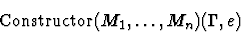is bidirectional. Similarly, if all the arguments Mi are backward-directional macetes then the compound macete is also backward-directional.
• Sound macete constructor: If M2,M3 are bidirectional, then the compound macete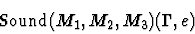is always bidirectional, regardless of M1. If M2 is backward-directional and M3 is bidirectional, then the compound macete is backward-directional. In all other cases, the compound macete is nondirectional.

# 14.4 Building Macetes

Schematic macetes are for the most part installed by the system when theorems installed.

1.
Elementary macetes are automatically installed when a theorem is added to a theory. The name of the installed macete is the same as the name of the theorem which generates it.
2.
Transportable macetes are installed when the transportable-macete modifier is present in the usage list of a theorem or axiom. Moreover, in this case, the name of the installed macete is the name of theorem which generates it with the prefix tr%.

Macetes are also automatically built when atomic sort and constant definitions are made by the user.

1.
For directly defined constants, the macete corresponding to the constant defining equation is installed. This macete is named
constant-name-equation_theory-name.
If the usage list of the definition includes the rewrite modifier and the defined constant is a function, the constant defining equation in applied form is also include as a macete named
constant-name-applied-equation_theory-name.
2.
For recursively defined constants, a number of macetes are installed:
(a)
The equation axioms named
constant-name-equation_theory-name
(b)
The minimality axiom named
definition-name-strong-minimality_theory-name
(c)
The minimality theorem named
definition-name-minimality_theory-name
3.
For defined atomic sorts a number of macetes are installed:
(a)
The sort defining axiom named
sort-name-defining-axiom_theory-name.
(b)
Some auxiliary theorems named
sort-name-in-quasi-sort_theory-name
and
sort-name-in-quasi-sort-domain_theory-name

Nondirectional schematic macetes usually are not associated to theorems. The form def-schematic-macete can be used for building schematic macetes of all kinds. You can build compound macetes using the form def-compound-macete.

# 14.5 Applicable Macetes

A macete is applicable'' to a formula if applying the macete modifies the formula in some way. The only sure-fire way of determining whether a macete is applicable, is to actually apply the macete and see the result. However, there are simple heuristic conditions which can be used to quickly determine that some macetes are not applicable. For example, for an elementary bidirectional macete, if the left hand side of the macete does not match any subexpression of the formula, then clearly the macete it is not applicable. For transportable macetes other similar inapplicability conditions are used. Macetes are tabulated in such a way that it is possible to quickly throw out inapplicable ones using these heuristic conditions. IMPS exploits this to provide users with a dynamic help facility for selecting macetes. The use of this facility is explained more extensively in Chapter 4.

# 14.6 Hints and Cautions

1.
Conceptually the macete mechanism is extremely simple. Users however, should not underestimate its power, usefulness, and flexibility. The utility of macetes hinges on three facts:
• The way a theorem is converted into a macete depends on the syntactic form of the the theorem as explained earlier in this section. In particular, it is important that theorems which are implications can be used as macetes along with those that are conditional equalities. Moreover, the conversion algorithm'' is based on a number of heuristics that in our experience work very well.
• Macetes can be combined using macete constructors. It has been our experience that carefully building a collection of compound macetes is an important part of developing a theory.
• Macetes are tabulated in such a way that the macetes which are applicable to a given formula can be retrieved very effectively and displayed to the user in a menu. In situations where over 500 macetes are installed, the menu usually contains less than twenty entries and takes less than ten seconds to compute.
2.
Though there are various modes of applying macetes, the default mode, with minor premises, is the one you want to use in most cases. The without-minor-premises macete constructor is often useful for building compound macetes to be used in this mode.Next: 15. The Iota Constructor Up: 2. User's Guide Previous: 13. Simplification